# Central Science: Chapter 16 Flashcards

Set Details Share
created 3 years ago by Chemistry4Life
2,342 views
College: First year, College: Second year, College: Third year, College: Fourth year
Subjects:
chemistry
Page to share:
Embed this setcancel
COPY
code changes based on your size selection
Size:
X

1

According to the Arrhenius concept, an acid is a substance that ________.
A) is capable of donating one or more H+
B) causes an increase in the concentration of H+ in aqueous solutions
C) can accept a pair of electrons to form a coordinate covalent bond
D) reacts with the solvent to form the cation formed by autoionization of that solvent
E) tastes bitter

B

2

A Br∅nsted-Lowry base is defined as a substance that ________.
A) increases [H+] when placed in H2O
B) decreases [H+] when placed in H2O
C) increases [OH-] when placed in H2O
D) acts as a proton acceptor
E) acts as a proton donor

D

3

A Br∅nsted-Lowry acid is defined as a substance that ________.
A) increases Ka when placed in H2O
B) decreases [H+] when placed in H2O
C) increases [OH-] when placed in H2O
D) acts as a proton acceptor
E) acts as a proton donor

E

4

Which one of the following is a Br∅nsted-Lowry acid?
A) (CH3)3NH+
B) CH3COOH
C) HF
D) HNO2
E) all of the above

E

5

A substance that is capable of acting as both an acid and as a base is ________.
A) autosomal
B) conjugated
C) amphiprotic
D) saturated
E) miscible

C

6

Which one of the following is a Br∅nsted-Lowry base?
A) (CH3)3N
B) CH3COOH
C) HF
D) HNO2
E) none of the above

A

7

The molar concentration of hydronium ion in pure water at 25 °C is ________.
A) 0.00
B) 1.0 × 10-7
C) 1.0 × 10-14
D) 1.00
E) 7.00

B

8

The molar concentration of hydroxide ion in pure water at 25 °C is ________.
A) 1.00
B) 0.00
C) 1.0 × 10-14
D) 1.0 × 10-7
E) 7.00

D

9

The magnitude of Kw indicates that ________.
A) water autoionizes very slowly
B) water autoionizes very quickly
C) water autoionizes only to a very small extent
D) the autoionization of water is exothermic

C

10

Which one of the following statements regarding Kw is false?
A) pKw is 14.00 at 25 °C.
B) The value of Kw is always 1.0 × 10-14.
C) Kw changes with temperature.
D) The value of Kw shows that water is a weak acid.
E) Kw is known as the ion product of water.

B

11

The hydride ion, H-, is a stronger base than the hydroxide ion, OH-. The product(s) of the reaction of hydride ion with water is/are ________.
A) H3O+ (aq)
B) OH- (aq) + H2 (g)
C) OH- (aq) + 2H+ (aq)
D) no reaction occurs
E) H2O2 (aq)

B

12

Of the following acids, ________ is a strong acid.
A) HNO2
B) H2CO3
C) HNO3
D) HClO
E) HF

C

13

Of the following acids, ________ is not a strong acid.
A) HNO2
B) H2SO4
C) HNO3
D) HClO4
E) HCl

A

14

Of the following, ________ is a weak acid.
A) HF
B) HCl
C) HBr
D) HNO3
E) HClO4

A

15

Which one of the following is the weakest acid?
A) HF (Ka = 6.8 × 10-4)
B) HClO (Ka = 3.0 × 10-8)
C) HNO2 (Ka = 4.5 × 10-4)
D) HCN (Ka = 4.9 × 10-10)
E) Acetic acid (Ka = 1.8 × 10-5)

D

16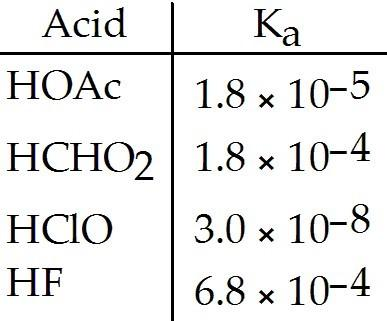Of the acids in the table below, ________ is the strongest acid.

A) HOAc
B) HCHO2
C) HClO
D) HF
E) HOAc and HCHO2

D

17

The Ka of hypochlorous acid (HClO) is 3.0 × 10-8 at . What is the percent ionization of hypochlorous acid in a aqueous solution of HClO at
A) 4.5 × 10-8
B) 14
C) 2.1 × 10-5
D) 0.14
E) 1.4 × 10-3

D

18

Classify the following compounds as weak acids (W) or strong acids (S):

benzoic acid, nitric acid, acetic acid

A) W W W
B) S S S
C) S W W
D) W S S
E) W S W

E

19

Classify the following compounds as weak acids (W) or strong acids (S):

hydrocyanic acid, hydrofluoric acid, hydrobromic acid

A) S W W
B) S S S
C) W W S
D) W S S
E) W S W

C

20

Classify the following compounds as weak acids (W) or strong acids (S):

hydrobromic acid, hydrochloric acid, hydrofluoric acid

A) W W W
B) S S S
C) S W W
D) W S S
E) S S W

E

21

Classify the following compounds as weak acids (W) or strong acids (S):

nitric acid, perchloric acid, chloric acid

A) W W W
B) W S S
C) S S S
D) S W W
E) W S W

C

22

Ammonia is a ________.
A) weak acid
B) strong base
C) weak base
D) strong acid
E) salt

C

23

HA is a weak acid. Which equilibrium corresponds to the equilibrium constant Kb for A-?
A) HA (aq) + H2O (l) ⇌ H2A+ (aq) + OH-(aq)
B) A- (aq) + H3O+ (aq) ⇌ HA (aq) + H2O (l)
C) HA (aq) + OH- (aq) ⇌ H2O (l) + H+ (aq)
D) A- (aq) + H2O (l) ⇌ HA (aq) + OH- (aq)
E) A- (aq) + OH- (aq) ⇌ HOA2- (aq)

D

24

A- is a weak base. Which equilibrium corresponds to the equilibrium constant Ka for HA?
A) HA (aq) + H2O (l) ⇌ H2A+ (aq) + OH- (aq)
B) A- (aq) + H3O+ (aq) ⇌ HA (aq) + H2O (l)
C) HA (aq) + H2O (l) ⇌ H3O+ (aq) + A- (aq)
D) A- (aq) + H2O (l) ⇌ HA (aq) + OH- (aq)
E) A- (aq) + OH- (aq) ⇌ HOA2- (aq)

C

25

Classify the following compounds as weak bases (W) or strong bases (S):

ammonia, fluoride ion, sodium ion

A) S S S
B) S W W
C) W W W
D) W S S
E) W S W

C

26

Classify the following compounds as weak bases (W) or strong bases (S):

methylamine, carbonate ion, potassium ion

A) S S S
B) S W W
C) W S S
D) W W W
E) W S W

D

27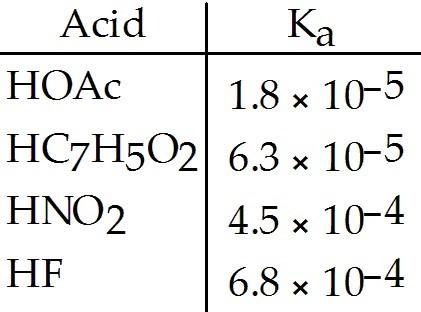Using the data in the table, which of the conjugate bases below is the strongest base?

A) OAc-
B) C7H5O2-
C) NO2-
D) F-
E) OAc- and C7H5O2-

A

28Using the data in the table, which of the conjugate bases below is the weakest base?

A) OAc-
B) C7H5O2-
C) NO2-
D) F-
E) OAc- and C7H5O2-

D

29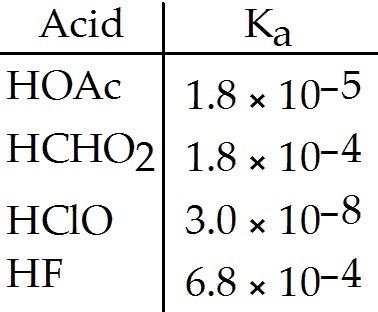Using the data in the table, which of the conjugate bases below is the weakest base?

A) OAc-
B) CHO2-
C) ClO-
D) F-
E) OAc- and CHO2-

D

30Using the data in the table, which of the conjugate bases below is the weakest base?

A) OAc-
B) CHO2-
C) ClO-
D) F-
E) OAc- and CHO2-

D

31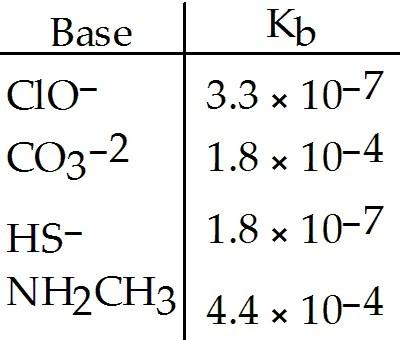Using the data in the table, which of the conjugate acids below is the strongest acid?

A) HClO
B) HCO3-
C) H2S
D) NH3CH3+
E) H2S and HClO

C

32Using the data in the table, which of the conjugate acids below is the strongest acid?

A) HClO
B) HCO3-
C) H2S
D) NH3CH3+
E) H2S and HClO

C

33Using the data in the table, which of the conjugate acids below is the weakest acid?

A) NH4+
B) C5H5NH+
C) H3NOH+
D) NH3CH3+
E) NH4+ and NH3CH3+

D

34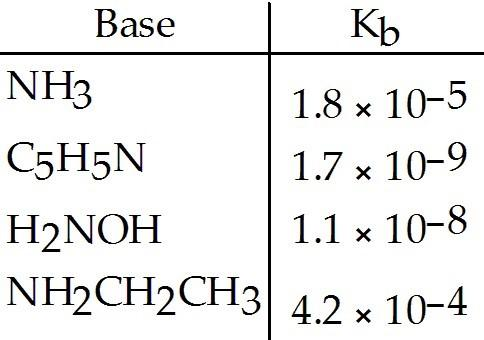Using the data in the table, which of the conjugate acids below is the weakest acid?

A) NH4+
B) C5H5NH+
C) NH3CH2CH3+
D) H3NOH+
E) NH4+ and NH3CH3+

C

35

Which of the following ions will act as a weak base in water?
A) OH-
B) Cl-
C) NO3-
D) ClO-
E) None of the above will act as a weak base in water.

D

36

Which of the following ions will act as a strong base in water?
A) HS-
B) F-
C) NO2-
D) ClO-
E) None of the above will act as a strong base in water.

E

37

Which of the following aqueous solutions has the highest [OH-]?
A) a solution with a pH of 3.0
B) a 1 × 10-4 M solution of HNO3
C) a solution with a pOH of 12.0
D) pure water
E) a 1 × 10-3 M solution of NH4Cl

D

38

Which of the following aqueous solutions has the lowest [OH-]?
A) a solution with a pH of 3.0
B) a 1 × 10-4 M solution of HNO3
C) a solution with a pOH of 12.0
D) pure water
E) a 1 × 10-3 M solution of NH4Cl

C

39

An aqueous solution of a particular compound has pH = 7.46. The compound is ________.
A) a weak base
B) a weak acid
C) a strong acid
D) a strong base
E) a salt

A

40

Of the following substances, an aqueous solution of ________ will form basic solutions.

NaHS, Cu(NO3)2, KHCO3, NaF

A) NaHS, Cu(NO3)2
B) KHCO3, NaHS
C) NaF only
D) NaF, KHCO3
E) NaHS, KHCO3 and NaF

E

41

Of the following substances, an aqueous solution of ________ will form basic solutions.

NH4Br, Pb(NO3)2, K2CO3, NaF

A) NH4Br, Pb(NO3)2
B) K2CO3, NH4Br
C) NaF only
D) NaF, K2CO3
E) NH4Br only

D

42

Of the compounds below, a 0.1 M aqueous solution of ________ will have the highest pH.
A) KCN, Ka of HCN = 4.0 × 10-10
B) NH4NO3, Kb of NH3 = 1.8 × 10-5
C) NaOAc, Ka of HOAc = 1.8 × 10-5
D) NaClO, Ka of HClO = 3.2 × 10-8
E) NaHS, Kb of HS- = 1.8 × 10-7

A

43

A 0.5 M solution of ________ has a pH of 7.0.
A) K2S
B) KF
C) KNO3
D) NH4Br
E) NaF

C

44

Which of the following acids will be the strongest?
A) H2SO4
B) HSO4-
C) H2SO3
D) H2SeO4
E) HSO3-

A

45

Of the following, which is the strongest acid?
A) HClO
B) HClO3
C) HClO2
D) HClO4
E) HIO

D

46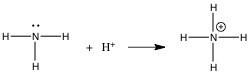In the gas phase reaction below, NH3 is acting as a(n) ________.

A) Br∅nsted-Lowry acid
B) Br∅nsted-Lowry base
C) Lewis base
D) Lewis acid
E) Arrhenius acid

C

47

What is the conjugate acid of NH2-?
A) NH2+
B) NH3+
C) NH4+
D) NH3
E) NH4OH

D

48

What is the conjugate acid of HCO3-?
A) CO22-
B) H2CO3
C) HCO22-
D) CO32-
E) none of the above

D

49

The conjugate acid of SO42- is ________.
A) OH-
B) H2SO4
C) HSO4-
D) HSO42-
E) H3SO4+

C

50

The conjugate base of HPO42- is ________.
A) PO43-
B) H2PO4
C) H3PO4
D) H2PO4-
E) none of the above

A

51

The conjugate base of HSO4- is ________.
A) H2SO4
B) HSO4+
C) H+
D) SO42-
E) HSO3+

D

52

The conjugate base of CH3NH3+ is ________.
A) CH3NH2+
B) CH3NH2-
C) CH3NH+
D) CH3NH2
E) none of the above

D

53

What is the conjugate acid of OH-?
A) O2
B) H2O
C) O-
D) O2-
E) H3O+

B

54

What is the pH of an aqueous solution at 25.0 °C that contains 2.50 × 10-4 M hydronium ion?
A) 10.4
B) 4.00
C) 2.50
D) 3.60
E) 7.00

D

55

What is the pOH of an aqueous solution at 25.0 °C that contains 2.50 × 10-4 M hydronium ion?
A) 3.60
B) 4.00
C) 2.50
D) 10.4
E) 7.00

D

56

What is the pH of an aqueous solution at 25.0 °C that contains 1.35 × 10-8 M hydroxide ion?
A) 7.87
B) 8.00
C) 6.13
D) 1.35
E) 7.00

C

57

What is the pOH of an aqueous solution at 25.0 °C that contains 1.35 × 10-8 M hydroxide ion?
A) 6.13
B) 7.87
C) 1.35
D) 8.00
E) 7.00

B

58

Calculate the pOH of a solution at 25.0 °C that contains 2.95 × 10-12 M hydronium ions.
A) 12.00
B) 2.95
C) 7.00
D) 2.47
E) 11.53

D

59

Calculate the pH of a solution at 25.0 °C that contains 2.95 × 10-12 M hydronium ions.
A) 2.95
B) 11.53
C) 7.00
D) 12.00
E) 2.47

B

60

Calculate the concentration (in M) of hydronium ions in a solution at 25.0 °C with a pOH of 3.58.
A) 2.63 × 10-4
B) 2.63 × 1010
C) 3.80 × 10-11
D) 3.80 × 103
E) 1.00 × 10-7

C

61

Calculate the concentration (in M) of hydroxide ions in a solution at 25.0 °C with a pOH of 3.58.
A) 2.63 × 1010
B) 3.80 × 10-11
C) 1.00 × 10-7
D) 3.80 × 103
E) 2.63 × 10-4

E

62

An aqueous solution contains 0.500 M NaOH at 25.0 °C. The pH of the solution is ________.
A) 0.500
B) 13.70
C) 0.301
D) 7.00
E) 13.50

B

63

An aqueous solution contains 0.390 M HCl at 25.0 °C. The pH of the solution is ________.
A) 0.41
B) 0.390
C) 13.61
D) 13.59
E) 7.00

A

64

HZ is a weak acid. An aqueous solution of HZ is prepared by dissolving 0.020 mol of HZ in sufficient water to yield 1.0 L of solution. The pH of the solution was 4.93 at 25.0 °C. The Ka of HZ is ________.
A) 1.2 × 10-5
B) 6.9 × 10-9
C) 1.4 × 10-10
D) 9.9 × 10-2
E) 2.8 × 10-12

B

65

The pH of a 0.55 M aqueous solution of hypobromous acid, HBrO, at 25.0 °C is 4.48. What is the value of Ka for HBrO?
A) 2.0 × 10-9
B) 1.1 × 10-9
C) 6.0 × 10-5
D) 3.3 × 10-5
E) 3.0 × 104

A

66

The pH of a 0.25 M aqueous solution of hydrofluoric acid, HF, at 25.0 °C is 2.03. What is the value of Ka for HF?
A) 2.0 × 10-9
B) 1.1 × 10-9
C) 6.0 × 10-5
D) 3.5 × 10-4
E) none of the above

D

67

The pH of a 0.60 M aqueous solution of formic acid, HCHO2, at 25.0 °C is 1.98. What is the value of Ka for formic acid?
A) 2.0 × 10-5
B) 1.8 × 10-4
C) 6.0 × 10-5
D) 3.5 × 10-4
E) none of the above

B

68

A 0.22 M aqueous solution of the weak acid HA at 25.0 °C has a pH of 4.15. The value of Ka for HA is ________.
A) 7.1 × 10-5
B) 3.2 × 10-4
C) 2.2 × 10-1
D) 2.3 × 10-8
E) 3.2 × 104

D

69

The Ka of hypochlorous acid (HClO) is 3.00 x10-8. What is the pH at 25.0 °C of an aqueous solution that is 0.0200 M in HClO?
A) +2.45
B) -2.45
C) -9.22
D) +9.22
E) +4.61

E

70

The Ka of acetic acid (HC2H3O2) is 1.8 x 10-5. What is the pH at 25.0 °C of an aqueous solution that is 0.100 M in acetic acid?
A) +2.87
B) -2.87
C) -11.13
D) +11.13
E) +6.61

A

71

The acid-dissociation constants of sulfurous acid (H2SO3) are Ka1 = 1.7 × 10-2 and Ka2 = 6.4 x 10-8 at 25.0 °C. Calculate the pH of a 0.163 M aqueous solution of sulfurous acid.
A) 4.53
B) 1.28
C) 1.86
D) 6.21
E) 1.93

B

72

The acid-dissociation constants of phosphoric acid (H3PO4) are Ka1 = 7.5 × 10-3, Ka2 = 6.2 x 10-8, and Ka3 = 4.2 × 10-13 at 25.0 °C. What is the pH of a 2.5 M aqueous solution of phosphoric acid?
A) 1.82
B) 0.40
C) 2.51
D) 0.86
E) 0.13

D

73

The pH of a 0.25 M aqueous solution ammonia, NH3, at 25.0 °C is 9.50. What is the value of Kb for NH3?
A) 2.5 × 10-1
B) 4.0 × 10-19
C) 4.0 × 10-9
D) 3.2 × 10-5
E) none of the above

C

74

A 0.10 M aqueous solution of the weak base B at 25.0 °C has a pH of 8.00. The value of Kb for B is ________.
A) 1.0 × 10-15
B) 1.0 × 10-11
C) 1.0 × 10-6
D) 1.0 × 10-12
E) none of the above

B

75

A 0.14 M aqueous solution of the weak acid HA at 25.0 °C has a pH of 3.15. The value of Ka for HA is ________.
A) 7.08 × 10-4
B) 3.58 × 10-6
C) 5.01 × 10-7
D) 7.02 × 10-8
E) none of the above

B

76

An aqueous solution of NaF is prepared by dissolving 0.350 mol of NaF in sufficient water to yield 1.0 L of solution. The pH of the solution was 8.93 at 25.0 °C. The Kb of F- is ________.
A) 1.2 × 10-5
B) 2.1 × 10-10
C) 6.9 × 10-9
D) 2.8 × 10-12
E) 9.9 × 10-2

B

77

The pOH of a 0.10 M solution of a weak base is 4.18. What is the Kb for this base?
A) 8.8 × 10-8
B) 2.1 × 10-4
C) 6.6 × 10-4
D) 4.4 × 10-8
E) 2.0 × 10-5

D

78

Determine the pH of a 0.35 M aqueous solution of CH3NH2 (methylamine). The Kb of methylamine is 4X4 x 10-4.
A) 10.00
B) 3.86
C) 12.09
D) 1.96
E) 13.24

C

79

An aqueous basic solution has a concentration of 0.050 M and Kb is 4.4 × 10-4. What is the concentration of hydroxide ion in this solution (M)?
A) 2.2 × 10-5
B) 4.5 × 10-3
C) 2.9 × 10-3
D) 4.7 × 10-3
E) 0.050

B

80

An aqueous basic solution has a concentration of 0.050 M and Kb is 4.4 × 10-4. What is the concentration of hydronium ion in this solution (M)?
A) 2.2 × 10-13
B) 2.2 × 10-12
C) 2.9 × 10-13
D) 0.050
E) 4.5 × 10-13

B

81

The acid-dissociation constant, Ka, for an unknown acid HA is 4.57 × 10-3. What is the base-dissociation constant, Kb, for the unknown anion A-?
A) 5.43 × 10-5
B) 4.57 × 10-3
C) 7.81 × 10-6
D) 2.19 × 10-12
E) 2.19 × 102

D

82

The base-dissociation constant, Kb, for an unknown base is 1.4 x 10-9. The acid-dissociation constant, Ka, for the conjugate ion is ________.
A) 1.0 × 10-7
B) 7.1 × 10-6
C) 1.4 × 10-23
D) 1.4 × 10-5
E) 7.1 × 10-4

B

83

The Ka for HCN is 4.9 × 10-10. What is the value of Kb for CN-?
A) 2.0 × 10-5
B) 4.0 × 10-6
C) 4.9 × 104
D) 4.9 × 10-24
E) 2.0 × 109

A

84

Ka for HF is 7.0 × 10-4. Kb for the fluoride ion is ________.
A) 2.0 × 10-8
B) 1.4 × 10-11
C) 7.0 × 10-18
D) 7.0 × 10-4
E) 1.4 × 103

B

85

Ka for HA is 4.9 × 10-10. What is the pH of a 0.068 M aqueous solution of A-?
A) 11.07
B) 0.74
C) 2.96
D) 7.00
E) 13.24

A

86

Ka for arsenic acic, HAsO42-, is 7.5 × 10-12. What is the pH of a 0.15 M aqueous solution of AsO43-?
A) 7.97
B) 12.10
C) 8.04
D) 1.96
E) 6.00

B

87

The pH of a 0.15 M aqueous solution of NaBrO (the sodium salt of HBrO) is 10.7. What is the Ka for HBrO?
A) 8.9 × 10-4
B) 1.6 × 10-6
C) 1.3 × 10-12
D) 3.3 × 10-8
E) 6.0 × 10-9

E

88

The conjugate base of NH3 is ________.
A) NH2-
B) NH4+
C) NH2OH
D) H3O+
E) OH-

A

89

The conjugate base of H2PO4- is ________.
A) H3PO4
B) HPO42-
C) PO43-
D) H3O+
E) OH-

A

90

In acidic solution, ________.
A) [H3O+] > [OH-]
B) [H3O+] = [OH-]
C) [H3O+] < [OH-]
D) [O ] > 7.00
E) [ ] = 0M

A

91

Which solution below has the highest concentration of hydroxide ions?
A) pH = 12.5
B) pH = 11
C) pH = 8.3
D) pH = 6.0
E) pH = 3.0

A

92

Which solution below has the highest concentration of hydronium ions?
A) pH = 3.0
B) pH = 10
C) pH = 7.0
D) pH = 6.4
E) pH = 11.2

A

93

An aqueous solution at 25.0°C contains [H+] = 0.085 M. What is the pH of the solution?
A) 1.07
B) -1.07
C) 13.0
D) 0.0850
E) 1.20 × 10-13

A

94

The pH of an aqueous solution at 25.0 °C is 10.55. What is the molarity of H+ in this solution?
A) 2.8 × 10-11
B) 3.5 × 10-4
C) 3.45
D) 1.1 × 10-13
E) 3.5 × 1010

A

95

Calculate the molarity of hydroxide ion in an aqueous solution that has a pOH of 3.00.
A) 1.0 × 10-3
B) 11.00
C) 1.0 × 10-11
D) 3.0 × 10-14
E) 1.1 × 10-13

A

96

What is the pH of an aqueous solution at 25.0 °C in which [H+] is 0.0015 M?
A) 6.50
B) 2.82
C) -2.82
D) -6.50
E) none of the above

B

97

What is the pOH of an aqueous solution at 25.0 °C in which [H+] is 0.0050 M?
A) 8.70
B) 11.70
C) -11.70
D) -8.70
E) none of the above

B

98

What is the pH of an aqueous solution at 25.0 °C in which [OH-] is 0.0030 M?
A) 5.81
B) -11.48
C) 2.52
D) -2.52
E) 11.48

E

99

An aqueous solution of ammonia at 25.0 °C has a [OH-] of 0.0010 M. What is the pOH of the solution?
A) 11.00
B) 3.00
C) -3.00
D) 6.91
E) -11.00

B

100

What is the concentration (in M) of hydronium ions in a solution at 25.0 °C with pH = 4.146?
A) 4.15
B) 9.85
C) 1.40 × 10-10
D) 7.15 × 10-5
E) none of the above

D

101

What is the concentration (in M) of hydroxide ions in a solution at 25.0 °C with pH = 4.282?
A) 4.28
B) 9.72
C) 1.92 × 10-10
D) 5.22 × 10-5
E) none of the above

C

102

An aqueous solution contains 0.10 M HNO3. The solution is ________.
A) acidic
B) basic
C) neutral
D) very dilute
E) highly colored

A

103

Which solution will be the most basic?
A) 0.10 M Ba(OH)2
B) 0.10 M KOH
C) 0.10 M H2O
D) 0.10 M CH3OH
E) All solutions have equal basicity.

A

104

A 1.0 × 10-2 M aqueous solution of at 25.0 °C has a pH of ________.
A) 12.30
B) 1.70
C) 2.0 × 10-2
D) 5.0 × 10-13
E) 12.00

A

105

What is the pH of a 0.030 M aqueous solution of calcium hydroxide?
A) 1.52
B) 12.48
C) 10.41
D) 12.78
E) 1.22

D

106

What is the pOH of a 0.030 M solution of calcium hydroxide?
A) 12.78
B) 1.22
C) 12.48
D) 10.41
E) 1.52

B

107

Hydrochloric acid is a strong acid. This means that ________.
A) HCl dissociates completely to H+(aq) and Cl-(aq) when it dissolves in water
B) HCl does not dissociate at all when it is dissolved in water
C) HCl produces a gaseous product when it is neutralized
D) HCl cannot be neutralized by a weak base
E) aqueous solutions of HCl contain equal concentrations of H+(aq) and OH-(aq)

A

108

The acid-dissociation constant at 25.0 °C for hypochlorous acid (HClO) is 3.0 × 10-8. At equilibrium, the molarity of H3O+ in a 0.066 M solution of HClO is ________.
A) 4.4 × 10-5
B) 0.066
C) 2.2 × 10-10
D) 4.35
E) 1.18

A

109

In which of the following aqueous solutions does the weak acid exhibit the highest percentage ionization?
A) 0.01 M H2SO3 (Ka = 1.4 × 10-2)
B) 0.01 M HCN (Ka = 6.2 × 10-10)
C) 0.01 M H2CO3 (Ka = 4.5 × 10-7)
D) 0.01 M HC3H5O2 (Ka = 1.3 × 10-5)
E) 0.01 M HOCl (Ka = 3.5 × 10-8)

A

110

In which of the following aqueous solutions does the weak acid exhibit the lowest percentage ionization?
A) 0.01 M HCN (Ka = 6.2 × 10-10)
B) 0.01 M H2CO3 (Ka = 4.5 × 10-7)
C) 0.01 M HNO2 (Ka = 4.0 × 10-4)
D) 0.01 M HCHO2 (Ka = 1.8 × 10-4)
E) 0.01 M HOCl (Ka = 3.5 × 10-8)

A

111

The of hypochlorous acid (HClO) is 3.0 × 10-8 at 25.0 °C. Calculate the pH of a 0.0385 M hypochlorous acid solution.
A) 3.05
B) 9.53
C) 4.47
D) 6.52
E) -3.05

C

112

The Ka of hydrofluoric acid (HF) at 25.0 °C is 6.8 × 10-4. What is the pH of a 0.45 M aqueous solution of HF?
A) 4.05
B) 1.76
C) 3.64
D) 0.35
E) 1.41

B

113

The of citric acid is 1.9 × 10-5 at 25.0 °C. What is the pH of a 0.35 M aqueous solution of citric acid?
A) 0.46
B) 2.59
C) 5.23
D) -3.46
E) 2.13

B

114

What is the [H+] (M) of a solution with a concentration of 1.4 x 10-2 M and Kb is 6.4 × 10-4 at 25.0 °C?
A) 3.3 × 10-12
B) 2.7 × 10-3
C) 3.0 × 10-3
D) 3.7 × 10-12
E) 11.43

D

115

Calculate the pH of a 0.500 M aqueous solution of N . The Kb of NH3 is 1.77 x 10-5.
A) 8.95
B) 11.47
C) 2.53
D) 11.77
E) 2.23

B

116

Which of the following 0.5 M aqueous salt solutions will have a pH of 7.0 at 25.0 °C?

LiF, RbBr, NaClO4, NH4Cl

A) LiF only
B) NaClO4 only
C) LiF and RbBr
D) RbBr and NaClO4
E) NH4Cl only

D

117

Which of the following salts will produce a basic solution?
A) Mg(ClO4)2
B) RbBr
C) Na2SO3
D) NH4NO3
E) KNO3

C

118

Which of the following salts will produce an acidic solution?
A) Sr(ClO4)2
B) KBr
C) NH4I
D) K2CO3
E) NaNO3

C

119

Which of the following salts will produce a neutral solution?
A) NaBr
B) LiNO2
C) LiF
D) Na2CO3
E) NH4Cl

A

120

The Ka of some acid, HA, at 25.0 °C is 4.9 × 10-10. What is the pH of a 0.050 M aqueous solution of A-?
A) 1.0 × 10-3
B) 3.00
C) 11.00
D) 9.9 × 10-12
E) 2.5 × 10-11

C

121

The Kb for A- is 4.9 x 10-10. What is the pOH of a 0.0727 M aqueous NaA solution at 25.0 °C?
A) 9.33
B) 5.22
C) 8.78
D) 1.14
E) 10.00

B

122

The Kb for A- is 4.9 x 10-10. What is the pH of a 0.0787 M aqueous NaA solution at 25.0 °C?
A) 9.33
B) 10.00
C) 5.21
D) 1.10
E) 8.79

E

123

The Ka for acid HA is 7.0 x 10-4. What is the pH of a 0.15 M aqueous solution of KA?
A) 0.82
B) 8.17
C) 5.83
D) 1.17
E) 5.01

B

124

The Ka for acid HA is 7.0 x 10-4. What is the pOH of a 0.10 M aqueous solution of KA?
A) 5.92
B) 1.00
C) 8.08
D) 1.58
E) 5.01

A

125

What is the pH of 0.626 M anilinium hydrochloride (C6H5NH3Cl) solution in water, given that Kb for aniline is 3.83 × 10-4?
A) 1.81
B) 5.39
C) 12.19
D) 12.42
E) 8.61

B

126

What is the pOH of 0.606 M anilinium hydrochloride (C6H5NH3Cl) solution in water, given that Kb for aniline is 3.83 × 10-4?
A) 12.42
B) 1.82
C) 8.60
D) 5.40
E) 12.18

C

127

What is the pH of a 0.40 M aqueous solution of NH4Br at 25.0 °C? Kb for NH3 is 1.8 × 10-5.
A) 4.82
B) 2.57
C) 9.18
D) 11.43
E) 11.23

A

128

What is the pOH of a 0.20 M aqueous solution of N Br at 25.0 °C? Kb for NH3 is 1.8 × 10-5.
A) 2.72
B) 4.98
C) 11.28
D) 11.23
E) 9.02

E

129

The Ka for formic acid (HCO2H) is 1.8 × 10-4. What is the pH of a 0.20 M aqueous solution of sodium formate (NaHCO2)?
A) 11.64
B) 5.48
C) 3.39
D) 8.52
E) 4.26

D

130

Of the following, which is the strongest acid?
A) HIO4
B) HIO3
C) HIO2
D) HIO
E) The acid strength of all of the above is the same.

A

131

Of the following, which is the weakest acid?
A) HPO3-
B) H3PO4
C) H2PO4-
D) HPO4-
E) The acid strength of all of the above is the same.

A

132

The pH of a 0.25 M aqueous solution of HA at 25.0 °C is 4.48. What is the value of Ka for HA?

4.4 × 10-9

133

The pOH of a 0.25 M aqueous solution of HA at 25.0 °C is 9.52. What is the value of Ka for HA?

4.4 × 10-9

134

A 0.045 M solution of ammonia is 2.0% ionized at 25.0 °C. The Kb at 25.0 °C for ammonia is ________.

1.8 × 10-5

135

What is the pH of a sodium fluoride solution prepared by adding 0.4198 grams of sodium fluoride to 100.0 ml of water at 25.0 °C? The Ka at 25.0 °C for HF is 7.2 × 10-4.

8.07

136

What is the pOH of a sodium fluoride solution prepared by adding 0.4198 grams of sodium fluoride to 100.0 ml of water at 25.0 °C? The Ka at 25.0 °C for HF is 7.2 × 10-4.

5.93

137

In the reaction

BF3 + F- → BF4-

BF3 acts as a Br∅nsted-Lowry acid.

false

138

An acid containing the COOH group is called a carbo-oxy acid.

false

139

A Lewis acid is an electron-pair acceptor, and a Lewis base is an electron-pair donor.

true